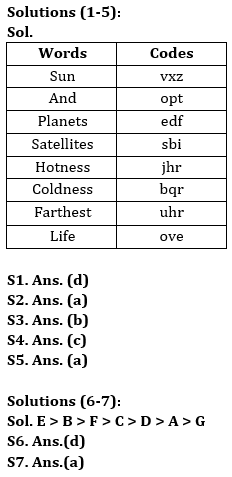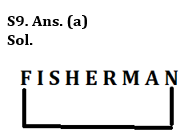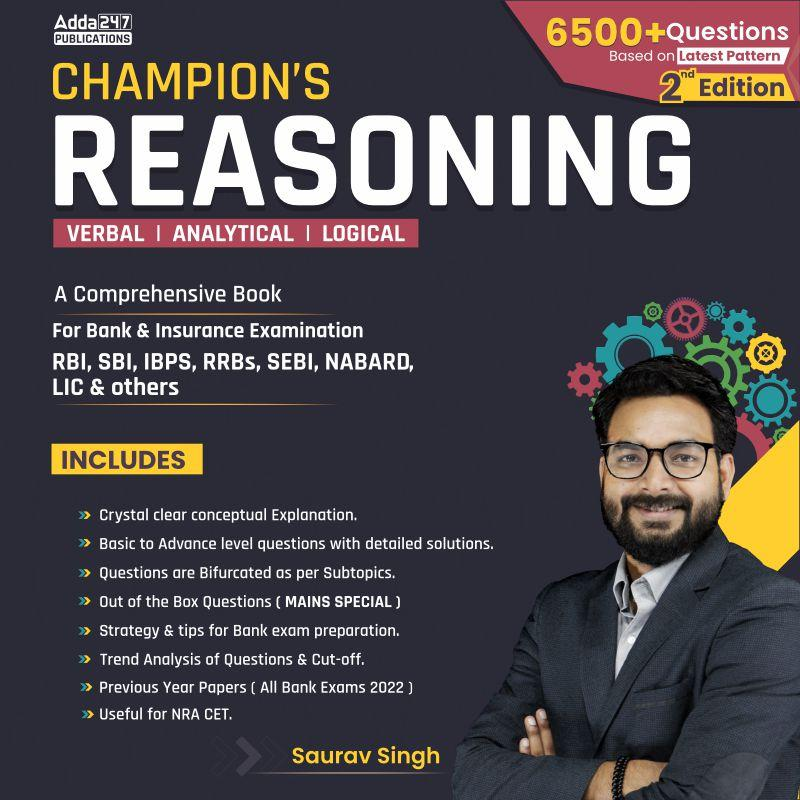Latest Banking jobs   »   IBPS reasoning

# Reasoning Quiz For IBPS PO Prelims 2023-02nd September

Directions (1-5): Answer the following question based on the information given below:
In a certain code language,
‘Sun and planets satellites’ is written as ‘vxz opt edf sbi’
‘Satellites sun hotness’ is written as ‘sbi vxz jhr’
‘Coldness and farthest sun’ is written as ‘bqr opt uhr vxz’
‘Farthest planets life’ is written as ‘uhr edf ove’

Q1. How is ‘hotness’ written in same language?
(a) sbi
(b) vxz
(c) edf
(d) jhr
(e) None of the above

Q2. Which of the following word is written as ‘vxz’?
(a) Sun
(b) Planets
(c) Farthest
(d) Satellites
(e) None of the above

Q3. How is ‘Farthest Life’ will be written in same language?
(a) opt edf
(b) uhr ove
(c) vxz edf
(d) ove bqr
(e) None of the above

Q4. Which of the following words are written as ‘sbi bqr’?
(a) Hotness satellites
(b) Sun planets
(c) Satellites coldness
(d) Planets coldness
(e) None of the above

Q5. How is ‘and planets’ written in same langauge?
(a) opt edf
(b) sbi uhr
(c) vxz ove
(d) ove opt
(e) None of the above

Directions (6-7): Answer the questions based on the information given below.
Ages of seven persons A, B, C, D, E, F and G are different. E is older than B who is older than F. Only G is younger than A. E is older than D who is just older than A. C is younger than F.

Q6. Who is immediate younger than B?
(a) A
(b) C
(c) D
(d) F
(e) None of the above

Q7. Who is the youngest person?
(a) G
(b) C
(c) D
(d) A
(e) None of the above

Q8. If 1 is subtracted from all the digits of the number ‘8368498934’, then what will be the sum of all the odd digits of the number thus formed after rearrangement?
(a) 30
(b) 32
(c) 28
(d) 36
(e) None of the above

Q9. How many pairs of letters are in the word ‘FISHERMAN’ which has as many letters between them in the word as in English alphabetical series (from both forward and backward)?
(a) One
(b) Four
(c) Two
(d) Thee
(e) None of the above

Q10. If it is possible to make a meaningful word from 3rd, 4th, 7th and 8th letters from the left end of the word “ONSTREAM”, then which will be the 3rd letter of the meaningful word? If no such word will be formed, mark the answer as ‘Y’. If more than one word can be formed mark the answer as ‘X’.
(a) X
(b) Y
(c) M
(d) T
(e) E

SolutionsS8. Ans. (b)
Sol. Given Number – 8368498934
After operation – 7257387823
So, required sum = 7 + 5 + 7 + 3 + 7 + 3 = 32S10. Ans. (a)
Sol. Given word – ONSTREAM
4th, 6th, 7th and 8th letters of word – T, E, A, M
Hence, more than one meaningful word can be formed i.e., TEAM, MEAT, MATE.## FAQs

### When will the IBPS PO prelims 2023 be conducted?

IBPS PO Prelims will be conducted on 23, 30 September, and 1 October 2023.

#### Congratulations!Union Budget 2023-24: Free PDF